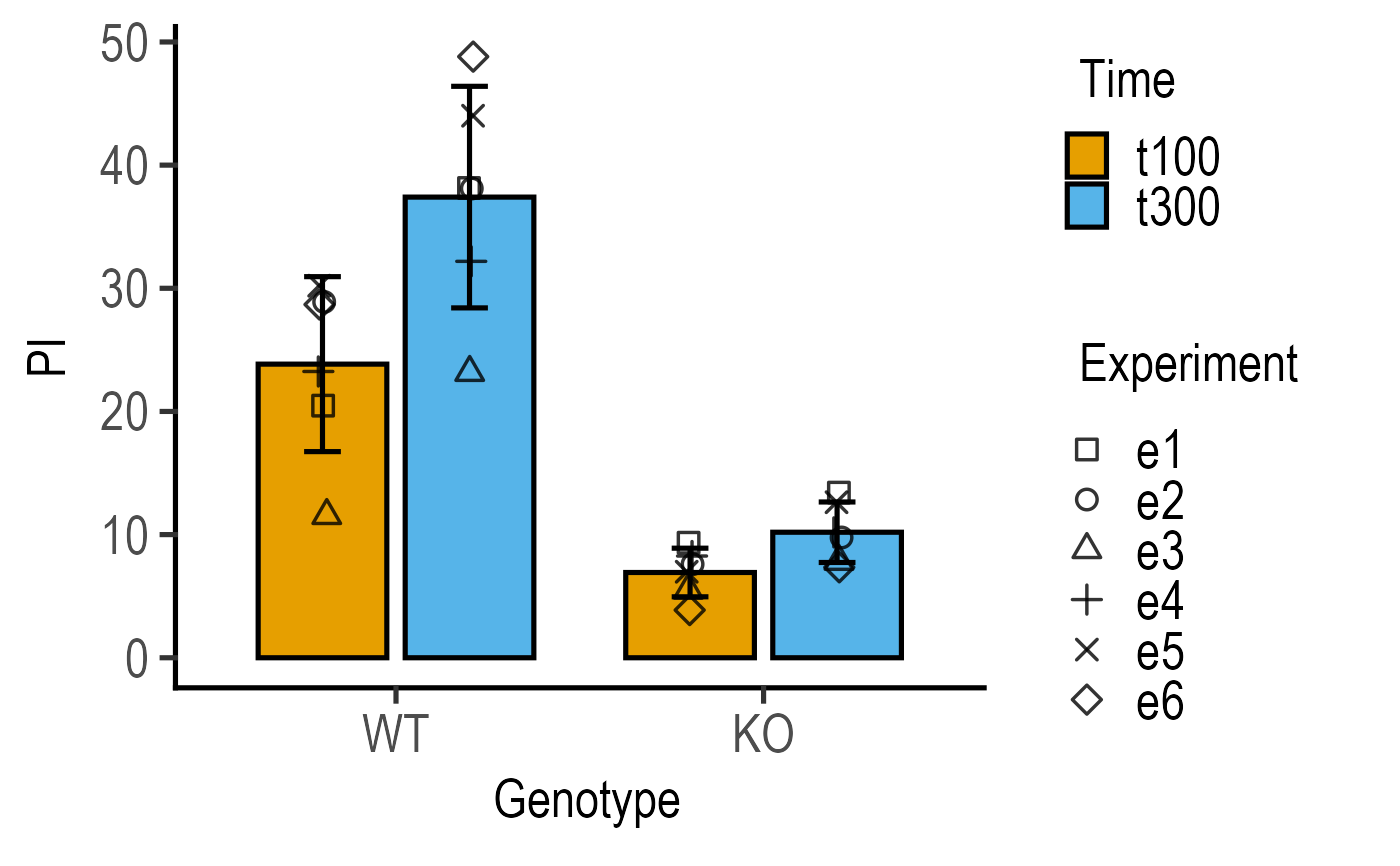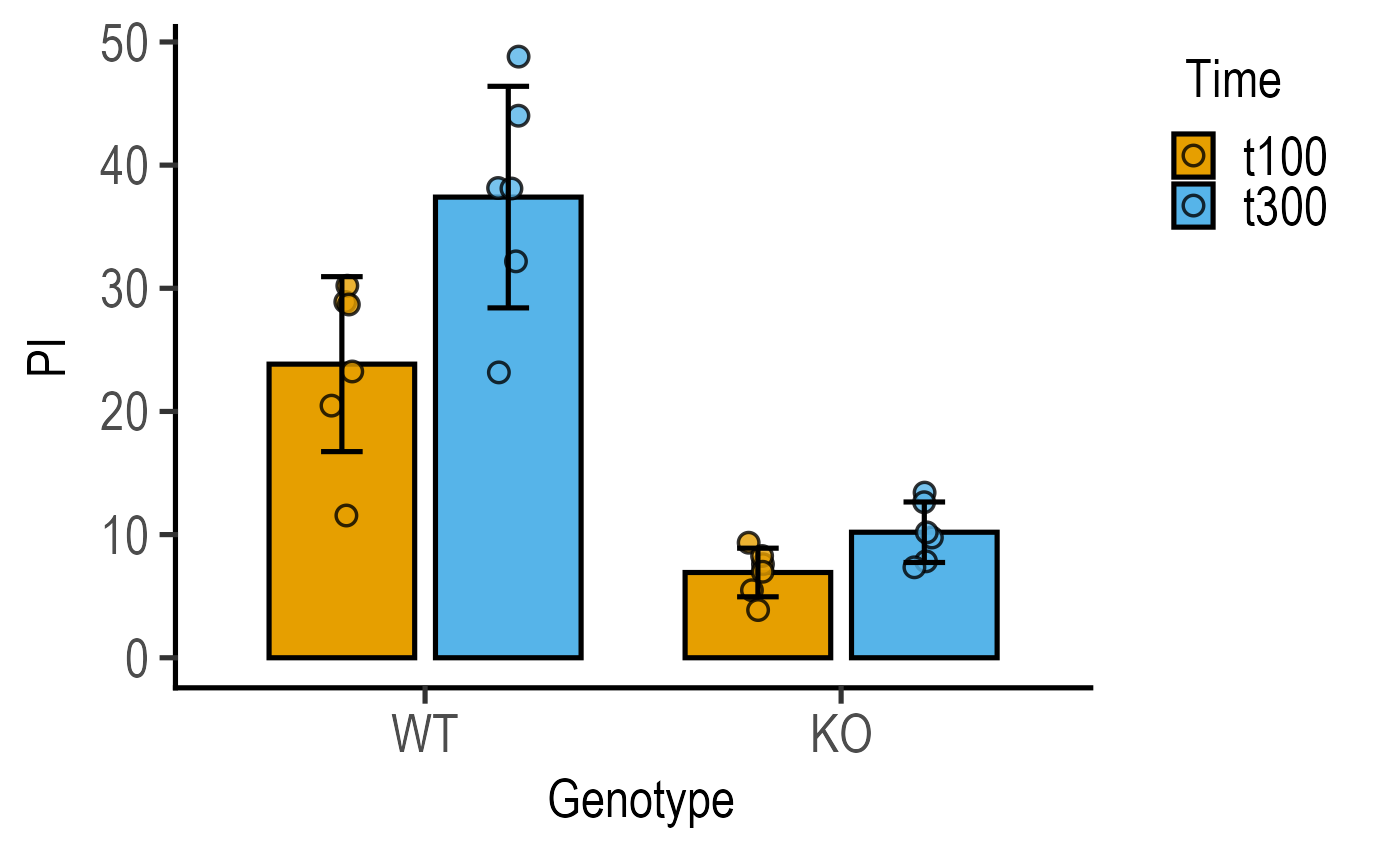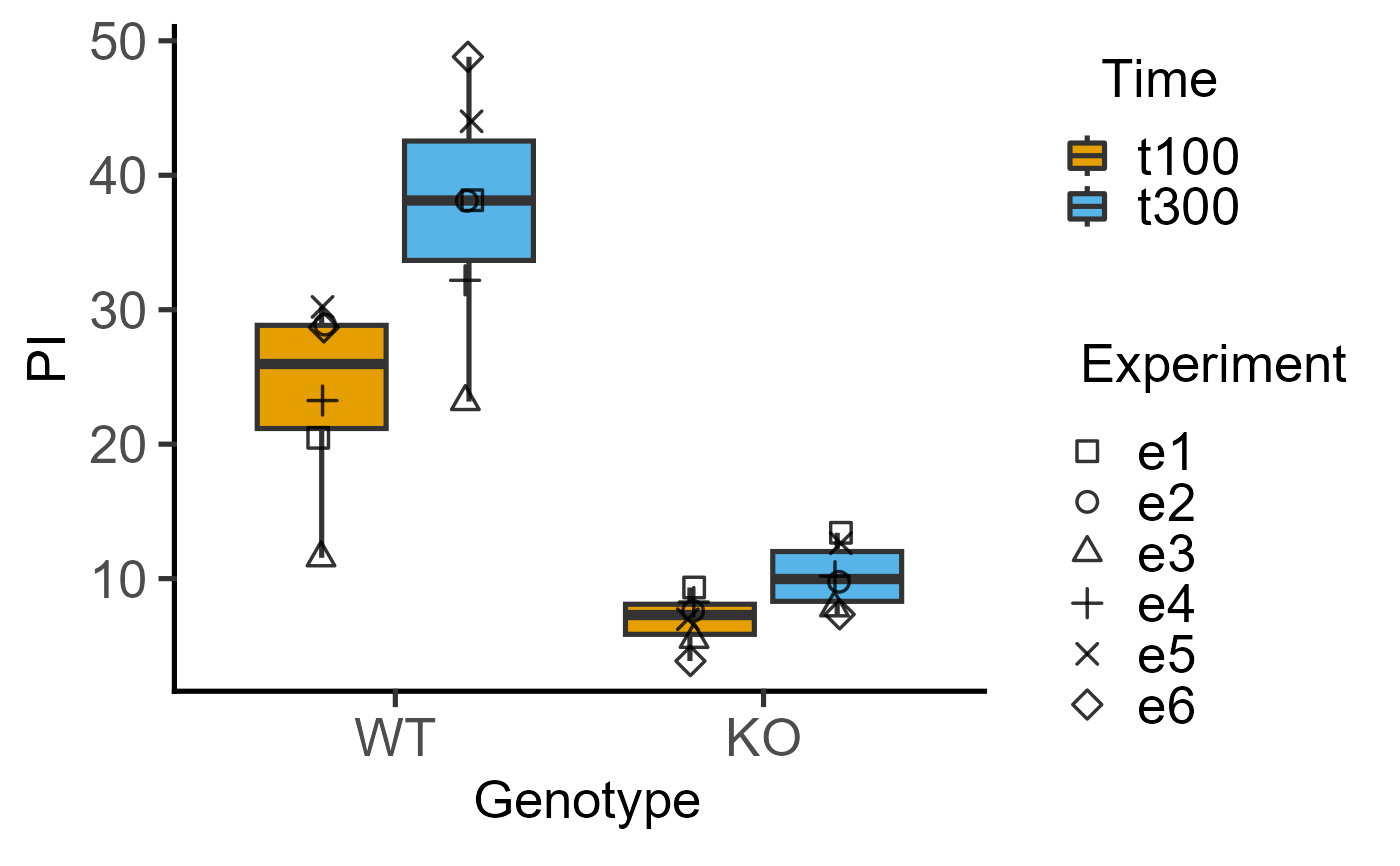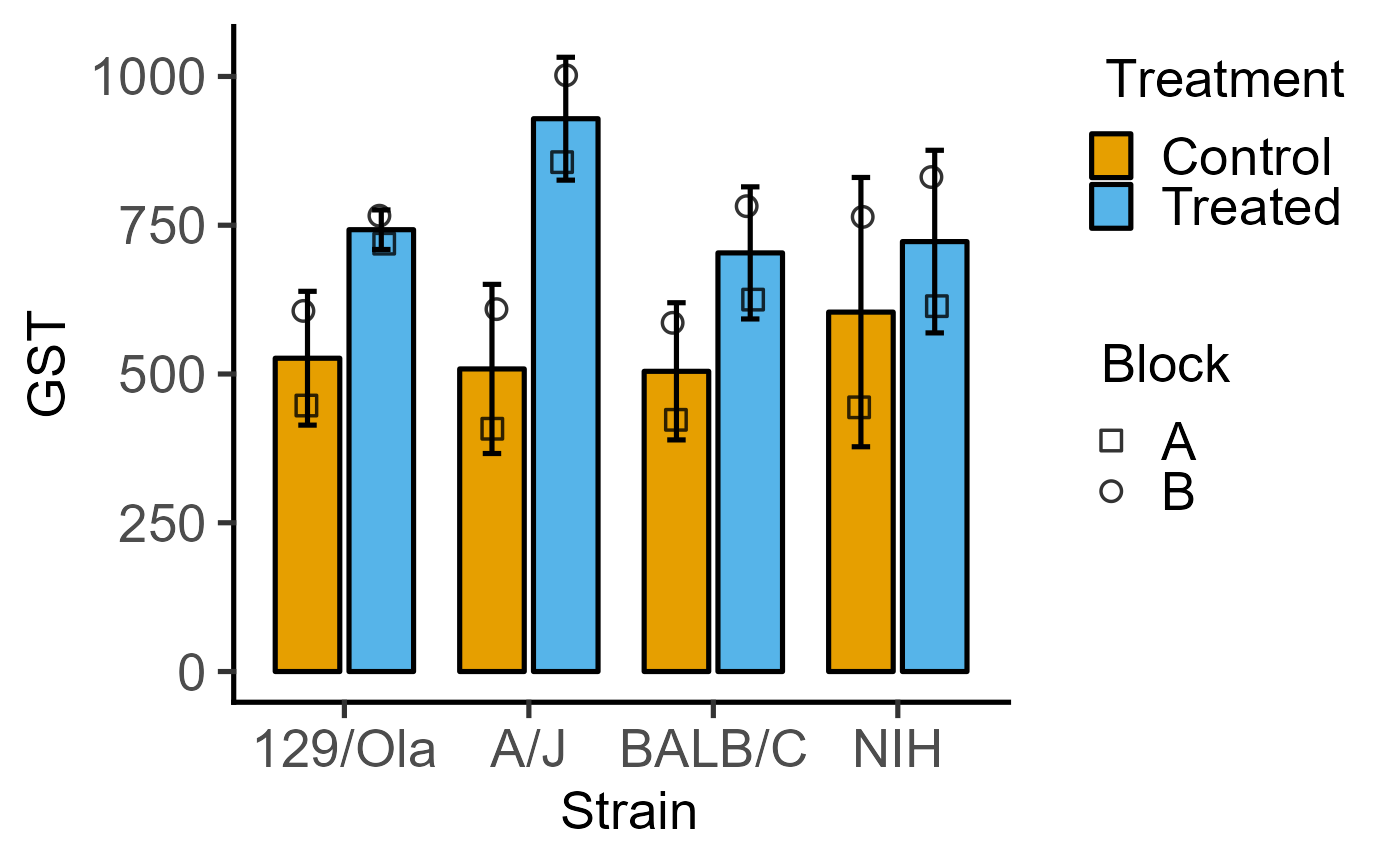The functions plot_3d_scatterbar, plot_3d_scatterbox, plot_4d_scatterbar and plot_4d_scatterbox are useful for plotting one-way or two-way ANOVA designs with randomised blocks or repeated measures. The blocks or subjects can be mapped to the shapes argument in both functions (up to 25 levels can be mapped to shapes; there will be an error if this number is exceeded). The 3d versions use the categorical variable (xcol) for grouping (e.g. one-way ANOVA designs), and 4d versions take an additional grouping variable (e.g. two-way ANOVA designs) that is passed to either boxes or bars argument.

plot_4d_scatterbar(
data,
xcol,
ycol,
bars,
shapes,
facet,
ErrorType = "SD",
symsize = 3,
s_alpha = 0.8,
b_alpha = 1,
bwid = 0.7,
jitter = 0.1,
ewid = 0.2,
TextXAngle = 0,
LogYTrans,
LogYBreaks = waiver(),
LogYLabels = waiver(),
LogYLimits = NULL,
facet_scales = "fixed",
fontsize = 20,
group_wid = 0.8,
symthick,
bthick,
ColPal = c("okabe_ito", "all_grafify", "bright", "contrast", "dark", "fishy", "kelly",
"light", "muted", "pale", "r4", "safe", "vibrant"),
ColRev = FALSE,
ColSeq = TRUE,
...
)

## Arguments

data

a data table, e.g. data.frame or tibble.

xcol

name of the column with the categorical factor to plot on X axis. If column is numeric, enter as factor(col).

ycol

name of the column to plot on quantitative variable on the Y axis.

bars

name of the column containing grouping within the factor plotted on X axis. Can be categorical or numeric X. If your table has numeric X and you want to plot as factor, enter xcol = factor(name of colum).

shapes

name of the column that contains matched observations, e.g. subject IDs, experiment ID.

facet

add another variable from the data table to create faceted graphs using ggplot2facet_wrap.

ErrorType

select the type of error bars to display. Default is "SD" (standard deviation). Other options are "SEM" (standard error of the mean) and "CI95" (95% confidence interval based on t distributions).

symsize

size of symbols, default set to 3.

s_alpha

fractional opacity of symbols, default set to 0.8 (i.e. 80% opacity). Set s_alpha = 0 to not show scatter plot.

b_alpha

fractional opacity of boxes. Default is set to 0, which results in white boxes inside violins. Change to any value >0 up to 1 for different levels of transparency.

bwid

width of boxes; default 0.7.

jitter

extent of jitter (scatter) of symbols, default is 0.1. Increase to reduce symbol overlap, set to 0 for aligned symbols.

ewid

width of error bars, default set to 0.2.

TextXAngle

orientation of text on X-axis; default 0 degrees. Change to 45 or 90 to remove overlapping text.

LogYTrans

transform Y axis into "log10" or "log2"

LogYBreaks

argument for ggplot2[scale_y_continuous] for Y axis breaks on log scales, default is waiver(), or provide a vector of desired breaks.

LogYLabels

argument for ggplot2[scale_y_continuous] for Y axis labels on log scales, default is waiver(), or provide a vector of desired labels.

LogYLimits

a vector of length two specifying the range (minimum and maximum) of the Y axis.

facet_scales

whether or not to fix scales on X & Y axes for all facet facet graphs. Can be fixed (default), free, free_y or free_x (for Y and X axis one at a time, respectively).

fontsize

parameter of base_size of fonts in theme_classic, default set to size 20.

group_wid

space between the factors along X-axis, i.e., dodge width. Default group_wid = 0.8 (range 0-1), which can be set to 0 if you'd like the two plotted as position = position_identity().

symthick

size (in 'pt' units) of outline of symbol lines (stroke), default = fontsize/22.

bthick

thickness (in 'pt' units) of bar and error bar lines; default = fontsize/22.

ColPal

grafify colour palette to apply, default "okabe_ito"; see graf_palettes for available palettes.

ColRev

whether to reverse order of colour within the selected palette, default F (FALSE); can be set to T (TRUE).

ColSeq

logical TRUE or FALSE. Default TRUE for sequential colours from chosen palette. Set to FALSE for distant colours, which will be applied using scale_fill_grafify2.

...

any additional arguments to pass to ggplot2stat_summary or ggplot2geom_point.

## Value

This function returns a ggplot2 object of class "gg" and "ggplot".

## Details

These functions rely on ggplot with geom_point and geom_bar (through stat_summary) or geom_boxplot geometries.

Variables other than the quantitative variable (ycol) will be automatically converted to categorical variables even if they are numeric in the data table.

Shapes are always plotted in black colour, and their opacity can be changed with the s_alpha argument and overlap can be reduced with the jitter argument. Other arguments are similar to other plot functions as briefly explained below.

Bars depict means using stat_summary with geom = "bar", fun = "mean" , and bar width is set to 0.7 (cannot be changed). Error bar width can be changed with the ewid argument.

Boxplot geometry uses geom_boxplot with position = position_dodge(width = 0.9), width = 0.6. The thick line within the boxplot depicts the median, the box the IQR (interquartile range) and the whiskers show 1.5*IQR.

In 4d versions, the two grouping variables (i.e. xcol and either boxes or bars) are passed to ggplot aesthetics through group = interaction{ xcol, shapes}.

Colours can be changed using ColPal, ColRev or ColSeq arguments. ColPal can be one of the following: "okabe_ito", "dark", "light", "bright", "pale", "vibrant, "muted" or "contrast". ColRev (logical TRUE/FALSE) decides whether colours are chosen from first-to-last or last-to-first from within the chosen palette. ColSeq (logical TRUE/FALSE) decides whether colours are picked by respecting the order in the palette or the most distant ones using colorRampPalette.

All four functions can be expanded further, for example with facet_grid or facet_wrap.

## Examples

#3d version for 1-way data with blocking
plot_3d_scatterbox(data = data_1w_death,
xcol = Genotype, ycol = Death, shapes = Experiment)#compare above graph to
plot_scatterbox(data = data_1w_death, xcol = Genotype, ycol = Death)#4d version for 2-way data with blocking
plot_4d_scatterbox(data = data_2w_Tdeath,
xcol = Genotype,
ycol = PI,
boxes = Time,
shapes = Experiment)plot_4d_scatterbar(data = data_2w_Festing,
xcol = Strain,
ycol = GST,
bars = Treatment,
shapes = Block)